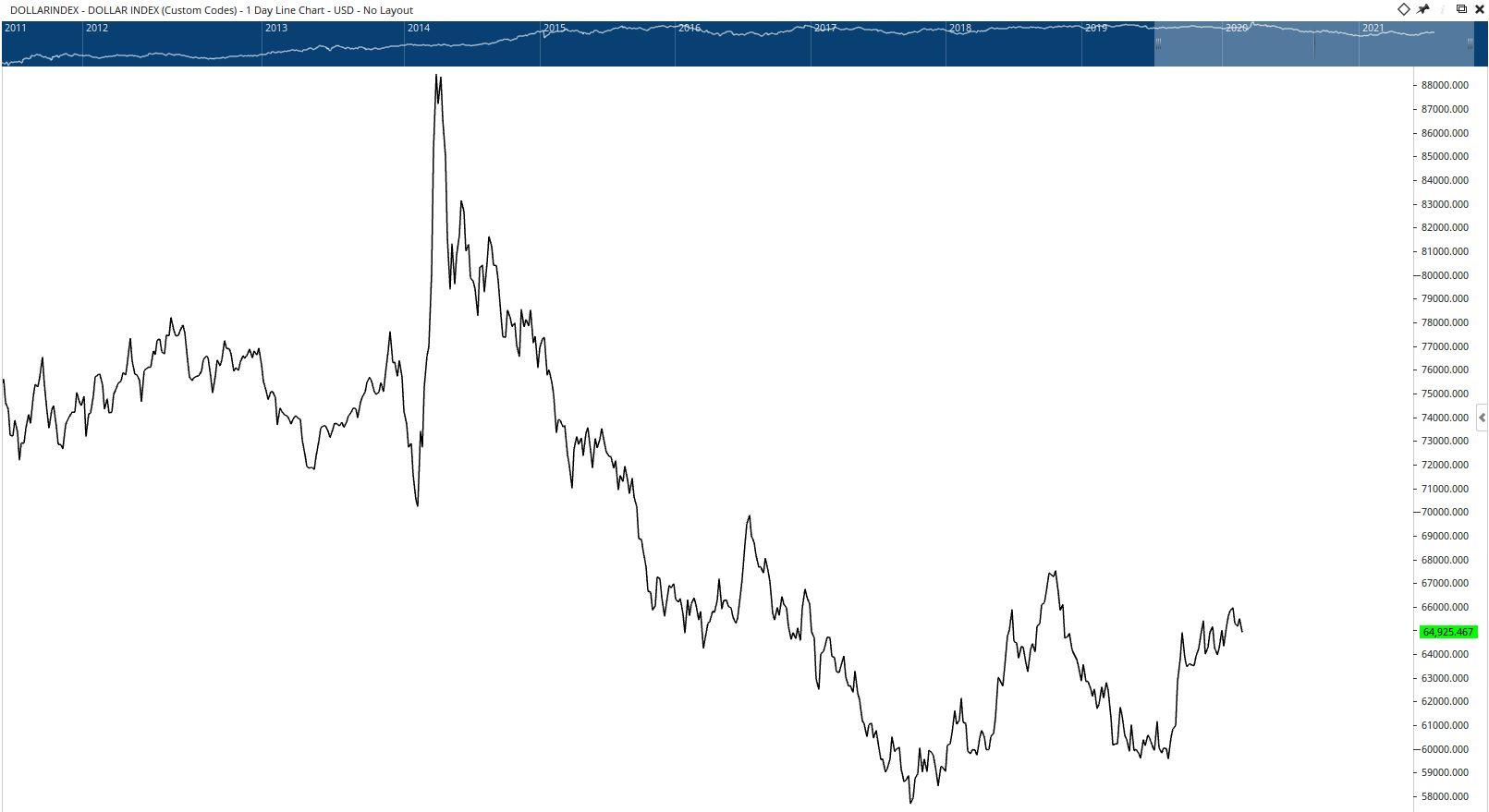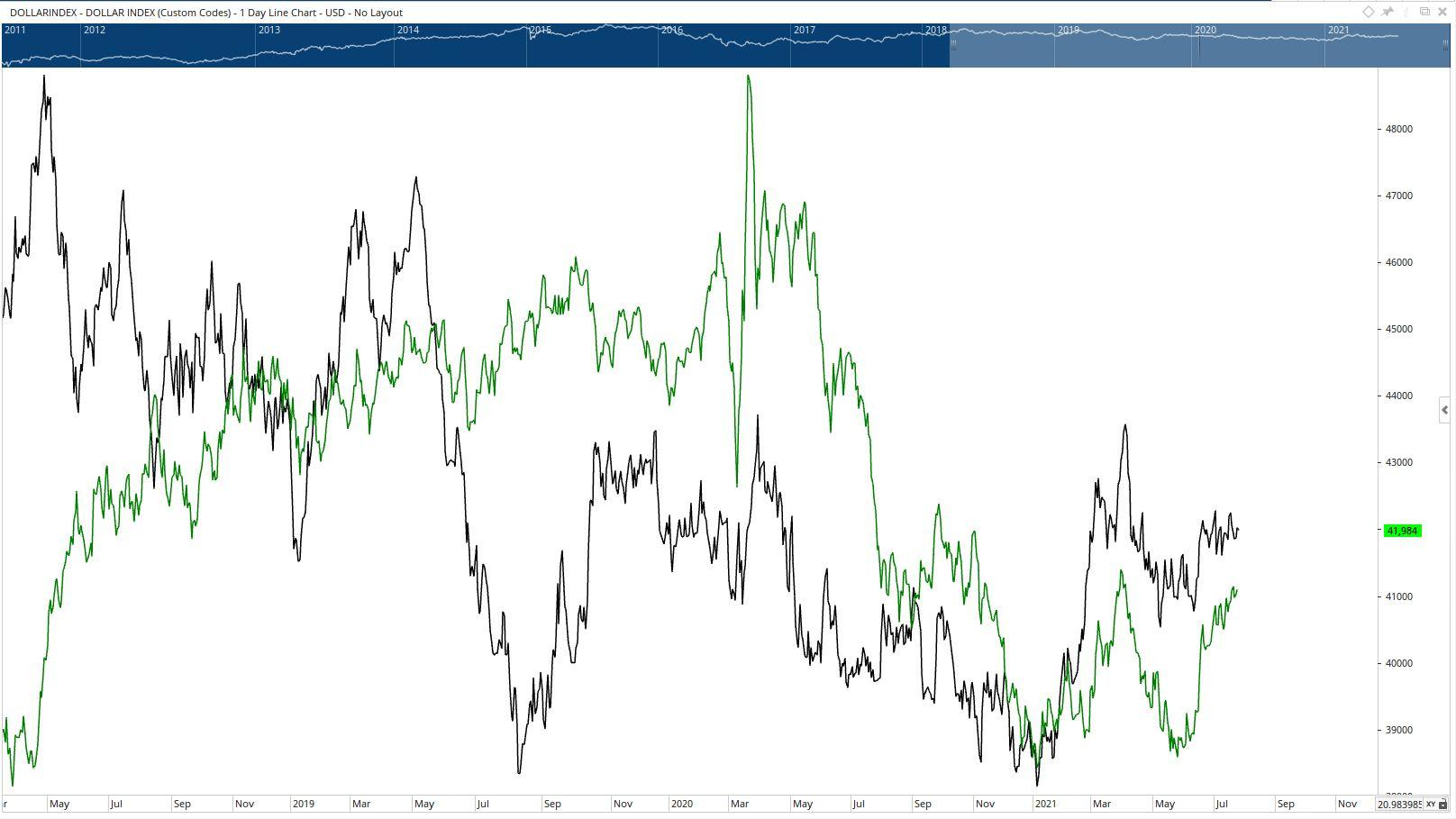Select Page

# Dollar Index Code (DXY)

Optuma Forums Optuma Scripting Dollar Index Code (DXY)

Viewing 5 posts - 1 through 5 (of 5 total)
• Author
Posts
• #65339

Hello Optuma friends,

I have been trying to create the “Custom Code” chart for the US Dollar Index (DXY) in order to get intraday feed through my FX broker instead of resorting to the ICE subscription . The equation seems simple enough, however, the Custom Code chart does not match the DXY chart.

According to several online references, the DXY is calculated using:
50.14348112 × EURUSD^-0.576 × USDJPY^0.136 × GBPUSD^-0.119 × USDCAD^0.091 × USDSEK^0.042 × USDCHF^0.036 (note that when the US dollar is not the base value in the currency cross, the value is negative).
The first factor, 50.14348112, is the historical value of the index to 100 on the date that the reference began.

I have tried the script:
50.14348112 * USDJPY:OF ^ 0.136 * USDCAD:OF ^ 0.091 * USDSEK:OF ^ 0.042 * USDCHF:OF ^ 0.036 * EURUSD:OF ^ -0.576 * GBPUSD:OF ^ -0.119
Resulting in (Notice the price scale):I have also tried parenthesis:
50.14348112 * (USDJPY:OF ^ 0.136) * (USDCAD:OF ^ 0.091) * (USDSEK:OF ^ 0.042) * (USDCHF:OF ^ 0.036) * (EURUSD:OF ^ -0.576) * (GBPUSD:OF ^ -0.119)
Resulting in (Black=Code, Green=DXY):Does anyone have any ideas on how to make this work? Am I missing something completely obvious?

Thank you all in advance.

Jeff

#65381

Hi Jeff,

We do not process the ^ in the formula. You’ll need to use the Power function.

For EUR  (EURUSD:OF ^ -0.576) use this instead

POWER(EURUSD:OF POWER=-0.576)

All the best

Mathew

#65383

Thank you for the continuous effort, Matthew! Unfortunately, this resulted in no chart being displayed at all. POWER(EURUSD:OF POWER=-0.576) worked well by itself, however, the combination did not.

50.14348112 * POWER(EURUSD:OF POWER=-0.576) * POWER(USDJPY:OF POWER=0.136) * POWER(GBPUSD:OF POWER=-0.119) * POWER(USDCAD:OF POWER=0.091) * POWER(USDSEK:OF POWER=0.042) * POWER(USDCHF:OF POWER=0.036)

#65387

Hi Jeffrey,

Where I restructured your code  as follows it produced a SHOWPLOT

Not sure though whether it the plot you are expecting as it comes up with some really extreme values. Give it a try.

Cheers

Trevor

#65397

Hi Jeffrey,

Where I restructured your code  as follows it produced a SHOWPLOT

Not sure though whether it the plot you are expecting as it comes up with some really extreme values. Give it a try.

Cheers

Trevor

Great Idea! But still not quite what I’m looking for. Thank you so much.

Viewing 5 posts - 1 through 5 (of 5 total)
• You must be logged in to reply to this topic.